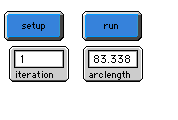WHAT IS IT?
------------

Helge von Koch was a Swedish mathematician who, in 1904 introduced what is now called the Koch curve.

Here is a simple geometric construction of the Koch curve.  Begin with a straight line.  This initial object is also called the "initiator."  Partition it into three equal parts.  Then replace the middle third by an equilateral triangle and take away its base.  This completes the basic construction step.  A reduction of this figure, made of four parts, will be used in the following stages.  It is called the "generator."  Thus, we now repeat, taking each of the resulting line segments and partitioning them into three equal parts, and so on.  The figure below illustrates this iterative process.

________________________	Step 0: "Initiator"

/\
/  \
/    \
/  	  \
_______/        \_______     Step 1: "Generator"

/\
__/  \__
\  	  /
/      \
___/\__/        \__/\___ 	Step 2

Self-similarity is built into the construction process.  Each part of the four parts in the k-th step is again a version scaled down by the factor of 3 of the entire curve in the previous (k-1)-st step.

The Koch curve is as difficult to understand as the Cantor set or the Serpinski tree.  However the problems with it are of a diffirent nature.  First of all -- as the name already expresses -- it is a curve, but this is not clear from the construction.  Secondly, this curve contains no straight lines which are smooth in the sense that we could see them as a carefully bent line.   Rather this curve has much of the complexity which we could see in a natural coastline: folds within folds within folds and so on.

Actually Koch's motivation for finding this curve was to provide another example for the discovery made by the German mathematician Karl Weierstrass, who in 1872 had precipitated a minor crisis in mathematics.  He had described a curve that could not be differentiated (did not have a tangent) at any of its points.  The ability to differentiate is central to differentable calculus and for a long time it was assumed that curves have tangent lines almost everywhere.

Let us now discuss the length of the Koch curve.  After the first iteration we have a curve which is made of four line segments of the same length, after the second iteration we will have each of the four segments broken into four more segments i.e. sixteen segments and so on.  After each iteration we increase the number of segments by the factor of four.  If we denote the number of segments after k-steps by S(k) then mathematically:

k
S(k) = 4

Now if the initial segment had length L the length of each of the four segments obtained after the first stage would be L/3.  After the second step the length of each of the sixteen segments is (L/3)/3 or L/9.  Denoting the length of each segment during the k-th iteration by L(k) we may write:

k
L(k) = L/(3 )

Multiplying the number of segments by the length of each segment we get the following expression for the length of the Koch curve after k steps of construction:

k
(4/3)

Clearly the length grows exponentially with the number of iterations so in fact the length of the entire Koch curve is infinite as is the arclength between any of its two points.  It therefore might come as a surprise that the area enclosed by the Koch curve is finite the proof of which we leave as an exercise for a motivated reader.

HOW TO USE IT
-------------

Reset the program by pushing the start button.  This will clear the graphics screen and initialize the globals.  Press repeatedly on the run button.  Each time you press the run button the construction algorithm is iterated and you will see successive approximations of the Koch curve.

THINGS TO NOTICE
----------------

To remove the middle third during each stage we simply change the color of the turtlle to black in the "move" procedure.  Then the turtle leaves no trace when it moves along the middle third.
Another thing to notice is how the "hatch" primitive is used to implement the iterative construction.

THINGS TO TRY
-------------------

You can combine three copies of the Koch curve to form a closed curve called the Koch snowflake.  Try to write a program that draws this fractal.

STARLOGOT FEATURES
------------------

Notice how the curves are formed using several agents following the same rules.  Also, take note of the use of the hatch command.